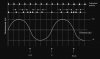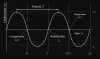# Physics: Post your doubts here!

#### Clark20

I have one question related to Dynamics. Suppose you throw a ball at an angle in air, or release it from rest so it falls under free-fall or you throw the ball upwards, there can be only 2 forces acting (air resistance and weight) on the ball?

Is there no type of applied force there in the downward direction which opposes air resistance when you throw and release the ball in the downward direction from top?

And an applied force in the direction of the object similarly which opposes air resistance in the case it is released at an angle? And even when you throw an object upwards, there is no applied force in the upward direction, but only weight and air resistance acting on the object?

(By applied force I mean the force along the direction in which the object is travelling.)

Last edited:

#### A*****

I have one question related to Dynamics. Suppose you throw a ball at an angle in air, or release it from rest so it falls under free-fall or you throw the ball upwards, there can be only 2 forces acting (air resistance and weight) on the ball?

Is there no type of applied force there in the downward direction which opposes air resistance when you throw and release the ball in the downward direction from top?

And an applied force in the direction of the object similarly which opposes air resistance in the case it is released at an angle? And even when you throw an object upwards, there is no applied force in the upward direction, but only weight and air resistance acting on the object?

(By applied force I mean the force along the direction in which the object is travelling.)
You are right that there are only 2 forces acting on the ball in any case i.e. the weight of the ball and air resistance. The weight ALWAYS acts downwards but air resistance acts in the OPPOSITE direction of the ball's motion.
When you release it from a point above ground, the ball moves downwards so the air resistance acts upwards and weight downwards.
When it is thrown upwards, both the weight and air resistance act downwards.
When thrown at an angle, weight acts downwards and resistance is changing direction every instant as the ball changes direction.

#### Clark20

You are right that there are only 2 forces acting on the ball in any case i.e. the weight of the ball and air resistance. The weight ALWAYS acts downwards but air resistance acts in the OPPOSITE direction of the ball's motion.
When you release it from a point above ground, the ball moves downwards so the air resistance acts upwards and weight downwards.
When it is thrown upwards, both the weight and air resistance act downwards.
When thrown at an angle, weight acts downwards and resistance is changing direction every instant as the ball changes direction.
Thank you

#### Clark20

Need help understanding this question.

#### Clark20

I did. Still I dont quite get it.

#### bhagwan dhakal

can i get help for q no 5 iv of 9702/23/m/j 16

#### Clark20

Can anyone explain this question?

#### Garry Soloan

Does anyone have notes for Communications Systems?

#### Ebrahim12

View attachment 64541 can
somebody pls help me put with this??
(i)
ratio of input to output = (64)(0.21) dB
ratio of output to noise = 25 dB

ratio of input to noise = 25 + 13.44 = 26.88 dB
Normally you would multiply ratios to combine them, but since the decibel is in a logarithmic scale you add the ratios.

(ii)
26.88 = 10 log(P/9.2μ)
P = 4.5x10^-3

#### Ebrahim12

The frequencies in the graph are F-f, F, F+f
where F is the freq of carrier wave and f is the freq of the signal wave

(i)
F = 909kHz
lambda = 330 m

(ii) and (iii)

Bandwidth is the range of frequencies used for transmission
In general, bandwidth = (F+f) - (F-f) = 2f

Bandwidth = 918 - 900 = 18 kHz
f = 9000 Hz

#### PlanetMaster

Hello, I would like about Q22 in the 9702/11/m/j/18 I want to ask for Why is the question B and not A?
View attachment 64548
Here is the examiner's report on the question
View attachment 64550
Hi Donenr,

Welcome to XtremePapers Community.

Let us look at the air molecules in their undisturbed positions and compare them as a sound wave passes by.If we plot displacement on the y-axis and distance on the x-axis, we get a sine wave.

If we plot a displacement-time graph for a single particle we see:This is true whether we have a longitudinal or transverse wave.

•